# Evaluation

## Comparison of CFD Calculations with Experiments

### LES versus experiment at shock generator angle of 8 degrees

Velocity fluctuations in a plane parallel to the wall evidence the presence of low and high velocity streaks that populates canonical boundary layers. After the separation (identified by the first dashed line), the size of turbulent structures in the spanwise direction significantly increases and further downstream the turbulence slowly relaxes toward its canonical state. This figure illustrates the fact that the simulation is capable of capturing most of the finest turbulent structures present in a supersonic boundary layer.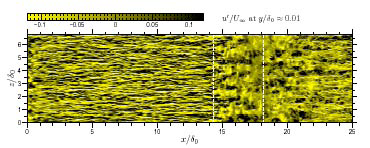Figure 5: Velocity fluctuations from LES in a plane parallel to the wall (corresponding to ${y^{+}\approx 12}$in the upstream boundary layer).

Quantitative comparisons in the symmetry plane are shown in Figure 6. The agreement between experiment and simulation is very good in the symmetry plane for the longitudinal velocity except in the separation bubble region. Nevertheless, it is important to mention that this region is very sensitive to the nature of inflow perturbations since a large variability of the results in this area has already been observed in the experiment, the 2006 data differing from the 2007 one, specifically in this region. The agreement with the experiment is also generally satisfactory for the Reynolds shear stress.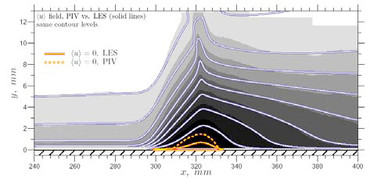|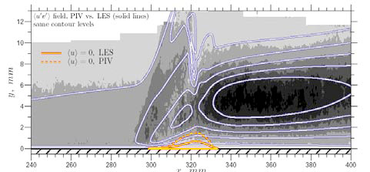Figure 6: Comparison of PIV2007 and LES results. Left: longitudinal velocity. Right: Reynolds shear stress.

Longitudinal evolution of turbulence spectra in the spanwise direction are presented in Figure 7 for both large and narrow span simulations. In the separation region, it appears that a large part of the energy is contained in the small wave numbers in the large span computation. The cutoff wave number imposed by the finite span is too large in the narrow span simulation. This forces the energy to concentrate at smaller scale and affects the results.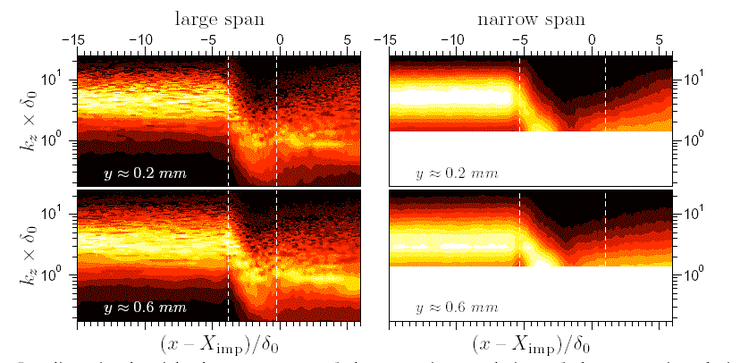Figure 7: Longitudinal evolution of turbulence spectra in the spanwise direction for two distances from the wall.

Low frequency movements of the reflected shock are clearly observed in Figure 8. As in the experiment the frequency of the power spectral density maximum is located at St=0.03. The agreement on the energy distribution between the narrow span computation and the experiment is very good.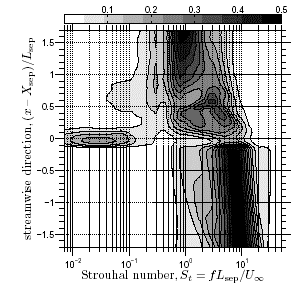|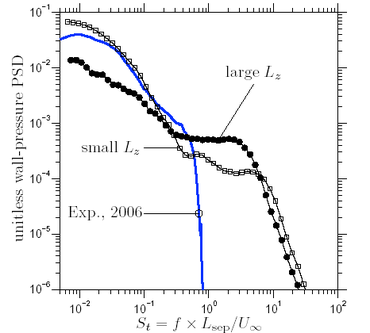Figure 8: Left: longitudinal evolution of LES pressure spectra in the streamwise direction. Right: pressure spectra at ${\left.X^{*}=0\right.}$### DES versus experiment at shock generator angle of 9.5 degrees

This section describes only the comparison of the SDES computation with the experiments. The reader is referred to Doerffer et al. (2010) for a presentation of RANS results.

The chosen technique of inflow turbulence generation is the Synthetic Eddy Method (SEM) (Garnier, 2009). Figure 9 illustrates clearly the fact that LES content (resolved eddies) is introduced at the entrance of the computational domain over the entire boundary layer height. Nevertheless, lateral boundary layers are treated in RANS mode.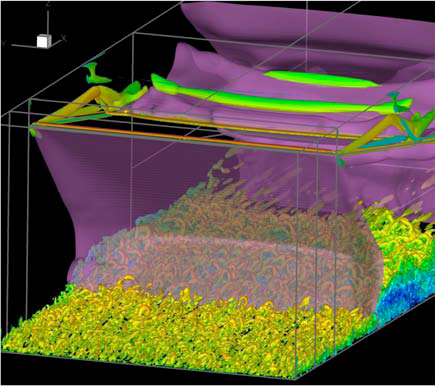Figure 9: Visualization of the flow (one isovalue of the Q criterion coloured by the longitudinal velocity). In purple, one isovalue of the pressure highlighting the incident shock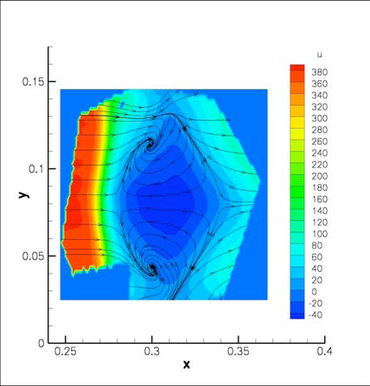|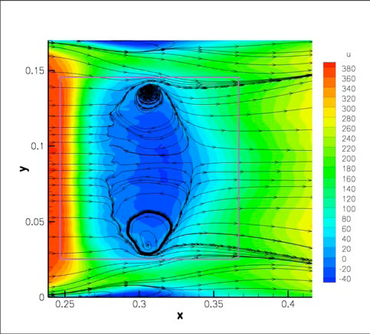Figure 10: Longitudinal velocity and pseudo streamlines in the plane located at 1.2 mm from the wall. Left PIV (IUSTI), right: SDES computation (ONERA).

It is found (Figure 10) that even if some improvement is observed with respect to RANS computations performed on the same grid (Doerffer et al. 2010), it seems that the extent of the predicted corner flows is too small. This is tentatively attributed to the fact that lateral walls are treated with RANS at the inflow.

The agreement between SDES and PIV is generally better in the symmetry plane (see Figure 11) even if the bubble aspect ratio is larger in the SDES computation than in the experiment. According to the model proposed in Piponniau et al. (2009), this should lead to an increase of the frequency of the reflected shock movement.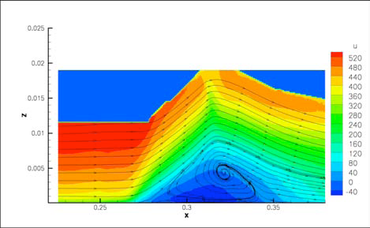|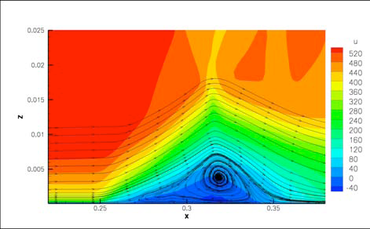Figure 11: Longitudinal velocity in the symmetry plane. Left: PIV; right: SDES

Even if the averaged results of this SDES simulation are far from being perfect, it is the only one that exists for the 9.5 degrees case carried out on the full geometry. Some work has then been performed to analyze the unsteady data hoping that it could complement the experimental results in a useful way.

Figure 12 presents isocontours of wall pressure fluctuations and streamlines which visualizes the flow topology. Streamlines should not be compared with those provided in Figure 10 since the flow topology changes drastically between the wall and 1.2 mm from the wall. Upstream of the interaction, pressure fluctuations are weak and they are due to turbulent fluctuations present in the boundary layer. A local pressure fluctuation maximum is observable near the separation at ${x=0.25}$. This quantity is used in the experiments to identify the beginning of the interaction zone. Nevertheless, it can be observed that the maxima of pressure fluctuations can be found in the corner flows and downstream from the interaction. In the latter case, these fluctuations are associated to Kelvin-Helmholtz type vortices which are generated in the shear layer above the separation bubble. More generally, these results indicate that the unsteady movements of highest intensity are localized in corner flows and a possible statistical link between these corner flows and the main separation area must be investigated.Figure 12: Wall pressure fluctuations and streamlines

The longitudinal evolution of the spectral energy density of wall pressure fluctuations premultiplied by the frequency and normalized by the signal variance is presented in Figure 13 and in Figure 14 for computation and the experiment. A frequency resolution FR of 200 Hz has been chosen to limit the statistical error due to short signal duration (only 80 ms).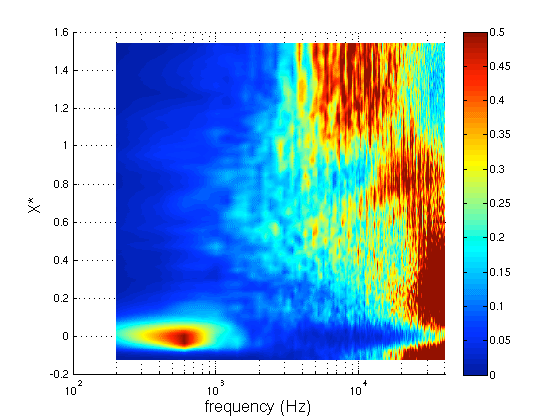Figure 13: Power spectral density of wall pressure fluctuations from SDES premultiplied by the frequency ${\left.(fG(f)/\sigma \right.}$in the plane ${\left.(f,X^{*}))\right.}$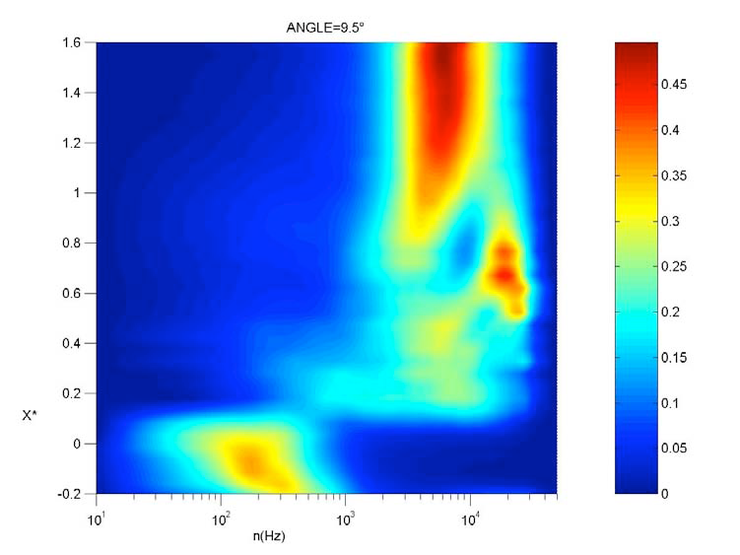Figure 14: Experimental power spectral density of wall pressure fluctuations premultiplied by frequency ${\left.nG(n)\right.}$in the plane ${\left.(n,X^{*})\right.}$, adapted from Dupont et al. 2006

Very high frequency fluctuations are present in the inflow boundary layer ${\left(X^{*}<-0.05\right)}$. In the range ${\left.-0.05, the energy is concentrated in a very low frequency band with an energy peak in the range 300-500 Hz. In the experiment, a bump centred around 200 Hz is evidenced in the pressure spectra. The fact that higher frequencies are found in the computation is surprising if one scales the Strouhal number of the low frequency movements on the interaction length. Nevertheless, in the IUSTI model (Piponniau et al. 2009), the frequency depends on the bubble aspect ratio, a larger aspect ratio (as in this computation) giving a higher frequency. In the range ${\left.0.4, the spectrum is really broadband and, for ${\left.X^{*}>0.8\right.}$, we can observe that energy is concentrated in the range 3-10 kHz as in the experiment. Such frequencies correspond to large Kelvin-Helmholtz type structures formed in the shear layer above the separation bubble. In the experiment, the pressure signals are filtered at 40 kHz. This can explain the discrepancies observed above this frequency between SDES and experiment.

The computation gives information about the frequency content of the pressure fluctuations in the whole flow. In particular, the relative contribution of frequencies lower than 1000 Hz with respect to total fluctuations is plotted in Figure 15 for the semi-plane ${y<0.085}$. It can be observed that low frequencies contribute for more than 70% of the total energy under the footprint of the reflected shock. In the separated zone, 20% of pressure fluctuations are attributable to low frequencies. This figure is in agreement with the experimental observations of Dupont et al. (2006). A slightly larger level (30%) of low frequency content is found in the corner flow shear layers.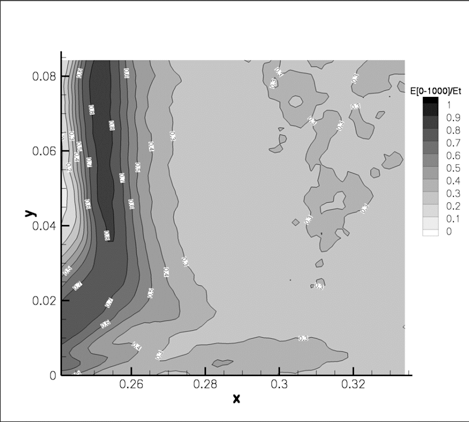Figure 15: Relative part of pressure fluctuations in the range 80-1000 Hz with respect to total fluctuation in the semi-plane ${y<0.085}$.

### Conclusions

The two studies reported in 2.1.1 (LES with periodic spanwise boundaries) and 2.1.2 (SDES of the full 3D interaction) are complementary and lead to conclusions as follows. Some of them are also reproduced in the Best Practice Advice.

• The low frequency mode can indeed be captured with simulation approaches.
• In LES with spanwise periodic boundary conditions attention needs to be placed in particular on the need for a sufficiently large domain size to capture both the mean flow and the low-frequency mode
• Grid dependency studies showed that grid size and by inference sub grid scale model characteristics are secondary factors for cases where the viscous sublayer is correctly resolved (wall modelling was not employed)
• For weak interaction cases the results for the spanwise periodic assumption compares favourably with experimental data.
• Strong interactions give highly three-dimensional interactions which require sidewall boundary layers to be included in the calculations.
• DES with additional inflow stimulation (i.e. SDES) provides a reference calculation.

Contributed by: Jean-Paul Dussauge (*), P. Dupont (*) , N. Sandham (**), E. Garnier (***) — (*) Aix-Marseille Université, and Centre National de la Recherche Scientifique UM 7343, (**) University of Southampton, (***) ONERA/DAAP, Meudon, France